Thermodynamics and Propulsion

6.5 Irreversibility, Entropy Changes, and Lost Work''

Consider a system in contact with a heat reservoir during a reversible process. If there is heat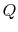absorbed by the reservoir at temperature, the change in entropy of the reservoir is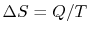. In general, reversible processes are accompanied by heat exchanges that occur at different temperatures. To analyze these, we can visualize a sequence of heat reservoirs at different temperatures so that during any infinitesimal portion of the cycle there will not be any heat transferred over a finite temperature difference.

During any infinitesimal portion, heatwill be transferred between the system and one of the reservoirs which is at. Ifis absorbed by the system, the entropy change of the system is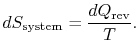The entropy change of the reservoir isThe total entropy change of system plus surroundings is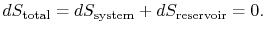This is also true if there is a quantity of heat rejected by the system.

The conclusion is that for a reversible process, no change occurs in the total entropy produced, i.e., the entropy of the system plus the entropy of the surroundings:.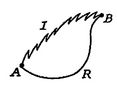We now carry out the same type of analysis for an irreversible process, which takes the system between the same specified states as in the reversible process. This is shown schematically in Figure 6.7, with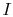anddenoting the irreversible and reversible processes. In the irreversible process, the system receives heat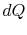and does work. The change in internal energy for the irreversible process isFor the reversible process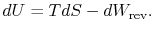Because the state change is the same in the two processes (we specified that it was), the change in internal energy is the same. Equating the changes in internal energy in the above two expressions yieldsThe subscript actual'' refers to the actual process (which is irreversible). The entropy change associated with the state change is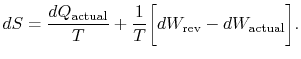(6..3)

If the process is not reversible, we obtain less work (see IAW notes) than in a reversible process,, so that for the irreversible process,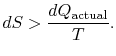There is no equality between the entropy change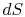and the quantityfor an irreversible process. The equality is only applicable for a reversible process.

The change in entropy for any process that leads to a transformation between an initial state a'' and a final state b'' is therefore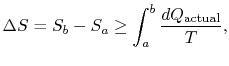where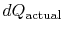is the heat exchanged in the actual process. The equality only applies to a reversible process.

The difference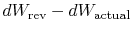represents work we could have obtained, but did not. It is referred to as lost work and denoted by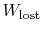. In terms of this quantity we can write,(6..4)

The content of Equation (6.4) is that the entropy of a system can be altered in two ways: (i) through heat exchange and (ii) through irreversibilities. The lost work (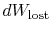in Equation (6.4)) is always greater than zero, so the only way to decrease the entropy of a system is through heat transfer.

To apply the second law we consider the total entropy change (system plus surroundings). If the surroundings are a reservoir at temperature, with which the system exchanges heat,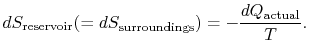The total entropy change is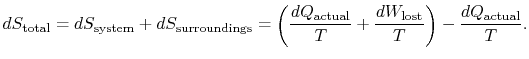The quantity (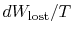) is the entropy generated due to irreversibility.

Yet another way to state the distinction we are making is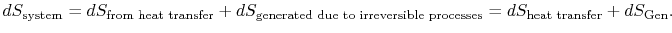(6..5)

The lost work is also called dissipation and noted. Using this notation, the infinitesimal entropy change of the system becomes: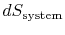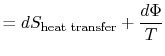orEquation (6.5) can also be written as a rate equation,(6..6)

Either of Equation (6.5) or (6.6) can be interpreted to mean that the entropy of the system,, is affected by two factors: the flow of heatand the appearance of additional entropy, denoted by, due to irreversibility6.1. This additional entropy is zero when the process is reversible and always positive when the process is irreversible. Thus, one can say that the system develops sources which create entropy during an irreversible process. The second law asserts that sinks of entropy are impossible in nature, which is a more graphic way of saying thatandare positive definite (always greater than zero), or zero in the special case of reversible processes.

The term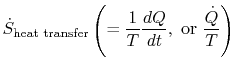which is associated with heat transfer to the system, can be interpreted as a flux of entropy. The boundary is crossed by heat and the ratio of this heat flux to temperature can be defined as a flux of entropy. There are no restrictions on the sign of this quantity, and we can say that this flux either contributes towards, or drains away, the system's entropy. During a reversible process, only this flux can affect the entropy of the system. This terminology suggests that we interpret entropy as a kind of weightless fluid, whose quantity is conserved (like that of matter) during a reversible process. During an irreversible process, however, this fluid is not conserved; it cannot disappear, but rather is created by sources throughout the system. While this interpretation should not be taken too literally, it provides an easy mode of expression and is in the same category of concepts such as those associated with the phrases flux of energy'' or sources of heat.'' In fluid mechanics, for example, this graphic language is very effective and there should be no objections to copying it in thermodynamics.

Muddy Points

Do we ever see an absolute variable for entropy? So far, we have worked with deltas only (MP 6.8)

I am confused as toas opposed to.(MP 6.9)

For irreversible processes, how can we calculateif not equal to?(MP 6.10)

UnifiedTP